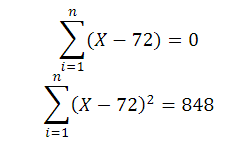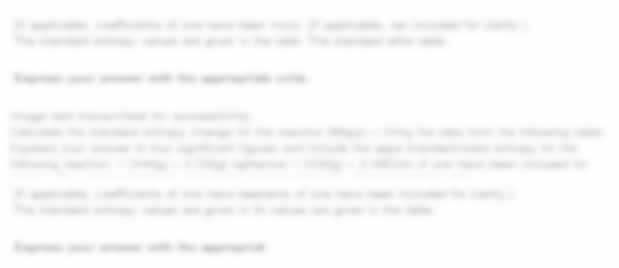UP TO 15 % DISCOUNT

Get Your Assignment Completed At Lower Prices

Plagiarism Free Solutions
100% Original Work
24*7 Online Assistance
Native PhD Experts
Hire a Writer Now
MTH220 Statistical Methods and Inference Assignment, SUSS, Singapore: Given that X follows a binomial model such that 𝑋 ~ 𝐵𝑖(2000,0.025), with the help of software
 University Singapore University of Social Science (SUSS) Subject MTH220 Statistical Methods and Inference Assignment
Posted on: 17th Oct 2023

# MTH220 Statistical Methods and Inference Assignment, SUSS, Singapore: Given that X follows a binomial model such that 𝑋 ~ 𝐵𝑖(2000,0.025), with the help of software

Question 1

(a) Given that X follows a binomial model such that 𝑋 ~ 𝐵𝑖(2000,0.025), with the help of software or otherwise, calculate 𝑃(𝑋  60). If you have used a software, state your methodology clearly.

(b) Compute 𝑃(𝑋  60) Use the normal approximation method and comment on your findings.

(c) Instead of analyzing the number of successes directly, another way of framing the question is to consider a proportion 𝑝̂ = 60 2000 = 0.03. Using the Central Limit Theorem, determine 𝑃(𝑝̂  0.03). By analyzing the proportion and with the help of the Central Limit Theorem, obtain an estimate for 𝑃(𝑋
 60) and show that it is equivalent to the normal approximation method in Question 1(b)

Question 2

A statistician with the Singapore Department of Statistics wants to determine the average annual income of people aged between 35 to 45 in Singapore. From last year’s reports, the mean annual income was \$50k SGD, with a standard deviation of \$5000.

(a) Calculate the required sample size so that the length of the 95% confidence interval of The mean Annual Income is less than \$1000.

(b) Also determine the required sample size if we want a 90% confidence interval instead.

(c) Discuss the application of Random Sampling, Systematic Sampling, and Convenience Sampling methods for the collection of annual income data, including the advantages and disadvantages of each method. Evaluate which method would be most suitable in terms of applicability and effectiveness.

##### Hire a Professional Essay & Assignment Writer for completing your Academic Assessments

Question 3

A researcher collects a dataset of 51 scores representing the performance of students on a mathematics test. The researcher wants to determine if the average score significantly differs from a population mean of 75. Apply a one-sample t-test to analyze the data and assess whether the average score is significantly different from the population mean at a significance level of
0.05. Perform the following tasks, you may use software such as Excel or R as a calculator, but full working must be shown otherwise.

(a) Provide the appropriate hypothesis statement.

(b) Calculate the t-statistic;

(c) 95% CI of the scores and calculate the p-value.

(d) Comment on the results in the context of the study.

(e) Show the code that will produce the entire t-test in R. Please google “t.test in R” if you need help.

Question 4

This is a continuation of Question 3. The research found some old records of past batches of students; however, the scores are recorded as summary statistics instead of individual scores. This is a summary of the data of students in a past batch:

X is the scores of the 54 students in the year 2021, and the overall scores can be summarized as follows:Apply a two-sample t-test of equal variance to analyze the data and assess whether the average score in 2021 is significantly different from the average score this year at a significant level of 0.01.

(a) Provide the appropriate hypothesis statements.

(b) Calculate the standard deviations and the pooled standard deviation.

(c) Calculate the test statistic and the critical region.

(d) Comment on the results in the context of the study.

Get Help By Expert

Are you a student at Singapore University of Social Science (SUSS) struggling with assignments like the MTH220 Statistical Methods and Inference Assignment? Look no further! We offer top-notch Tutor Marked Assignments (TMA) and individual assignment assistance tailored to SUSS students. Our best assignment help Singapore services ensure that you get the support you need to excel in your academic journey. From Essay Writing Services to specialized assistance like the MTH220 assignment you mentioned, our experts are here to guide you. Let us help you succeed in your courses at SUSS.

Categories:-##### No Need To Pay Extra
• Turnitin Report

\$10.00

\$9.00
Per Page
• Consultation with Expert

\$35.00
Per Hour
• Live Session 1-on-1

\$40.00
Per 30 min.
• Quality Check

\$25.00
##### Free

New Special Offer

## Get 30% Off

UP TO 15 % DISCOUNT

Get Your Assignment Completed At Lower Prices

Plagiarism Free Solutions
100% Original Work
24*7 Online Assistance
Native PhD Experts
Hire a Writer Now
My Assignment Help SG Services
My Assignment Help SG